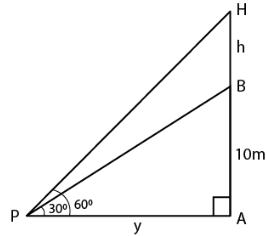Guru

# From a point P on the ground, the angle of elevation of the top of a 10 m tall building and a helicopter hovering over the top of the building are 300 and 600 respectively. Find the height of the helicopter above the ground.

• 0

sir this is the question from the book -ML aggarwal( avichal publication) class 10th , chapter20 , heights and distances
this is a very important question and has been asked in previous year exams.
we have been given that From a point P on the ground,
the angle of elevation of the top of a 10 m tall building
and a helicopter hovering over the top of the building are 300 and 600 respectively.
Find the height of the helicopter above the ground.

question no 15 , heights and distances , ICSE board

Share

1. Consider AB as the building and H as the helicopter hovering over it

P is a point on the ground

Angle of elevation of the top of building and helicopter are 300 and 600We know that

Height of the building AB = 10 m

Take PA = x m and BH = h m

In right triangle ABP

tan θ = P/B

Substituting the values

tan 300 = AB/PA = 10/x

So we get

1/√3 = 10/x

x = 10√3 m

In right triangle APH

tan 600 = AH/PA

tan 600 = (10 + h)/ x

So we get

√3 = (10 + h)/ 10√3

By further calculation

10√3 × √3 = 10 + h

30 = 10 + h

h = 30 – 10 = 20

Height of the helicopter from the ground = 10 + 20 = 30 m

• 0